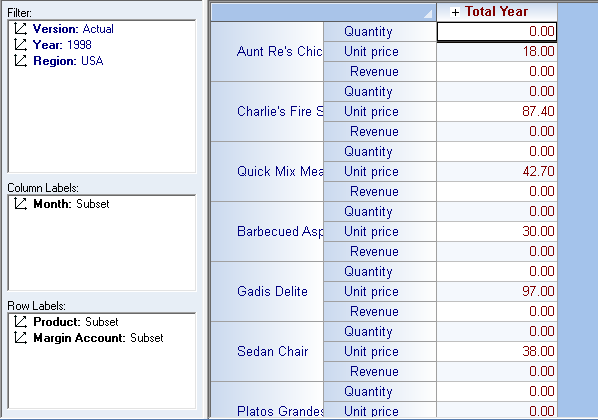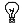## Import Fact DataThe Fact Data import process is where a potential difference in dimensionality really comes into play. After all, there may be Member intersection points in the import file (e.g., for the Region Canada, Unit Cost, 1998, for Products X, Y and Z) that do not exist in the database into which you intend to import the Fact Data (for example, if Canada is not a Member of the Region dimension in the database). On the other hand, there may exist a Dimension in the database (for example, Version) that does not exist in the import script-here you will have to decide, what Member of the Version dimension should the data be made to represent when it is imported?

We will consider some of these issues in the following example, which involves importing a previously created Cube Fact Data export file, 'Margin-Actual Unit Cost and Price.txt', into the database created in the previous steps, TestImp.olp.

In order to import Fact Data, you must have a database open, and a Cube must already exist-logically, you will have many of the Meta Data parameters set up beforehand, otherwise it would make no sense to import figures!

1.   From the PowerOLAP ribbon, go to Data tab, then in the Import control group select Factdata icon or Import Data command.
The Import Data dialog box is displayed:2.   Click Browse to select the file to import.

3.   Select the <file> you want to import
(for this example, Margin-Actual Unit Cost and Price.txt).

4.   Click Open.

5.   In the Import Data dialog box, select a Delimiter (e.g., Tab).

6.   Click OK. (There will be a message if the file is invalid.
The Import Fact Data dialog box appears:We will consider this dialog box in detail:

Located at the top right of the Import Data dialog box is a text box to select the Cube into which to import the data. All Cubes for the open database are listed. (In the example, there is a Margin cube in the database.)

7.   Select a Cube (E.g., Margin).

Note the Dimensions list box on the left.

PowerOLAP automatically compares the Dimensions in the import file to those in the chosen Cube and places an icon to the left of the Dimension name as follows:The Dimension is present in both the Cube and the import file.The Dimension is found in the Cube but not the import file.The Dimension is found in the import file but not the Cube.

Often, if you are importing Fact Data, there will be an exact match (the first icon)-because you intend to import Fact Data into a database that is set up to precisely "contain" the data in the import script. As in the example that we have, the import file has the same dimensionality as with the destination cube where we intend to import the Fact Data. Both the import file and the cube contain the dimensions: Version, Year, Region, Product, Month and Margin Account.

But there will be instances when there are no exact matches of Dimensions-the so-called "difference in dimensionality." (Thus, if there is a Product Account dimension that exists in the cube but not in the import file, then that dimension will be preceded by the second icon.)

It is therefore helpful to bear these things in mind:

a. For Dimension(s) that exist both in the cube and the import file
- You can choose to import data to populate individual, multiple or All Members, which is the default

b. For Dimension(s) found in the Cube but not the import file
- You may need to decide what Member in the Cube should be the receptor of the data-which Member logically best describes the data being imported.

c. For Dimension(s) that exist in the import file but not in the cube
- You may want to consider what Member should the data that will be brought over into the cube should come from. This is because we can only bring over one Member's data, if the method selected is 'Set'.

For Dimensions that exist in both the import file and a Cube, you can choose to import data to populate individual, multiple or All Members, which is the default.

For our example, since both the cube and import file have the same dimensionality, choose to import 'All'.

8.   In the Method section of the Import Fact Data dialog, select from the options: Set, Accumulate and Clear (For this example, select Set).

A description of what each option does is detailed at the end of this topic.

9.   Click OK.

The import file would process and you will be prompted with a message indicating the number of data points that have been successfully imported.10. Click OK to return to the main application window.

You can create a slice out of the Margin cube to check the fact data that was imported into the cube.After you import (or by any other means, enter) new data into your database, be sure to save the database changes to disk.

11. Select File button, Save Database.ImportantIf you import a text file that includes data for Aggregate Members, you will receive a message indicating that a number of lines COULD NOT be imported-referring to the Aggregate Members in the Cube.  You cannot import data into cells with aggregate information. In the created error log, the Aggregate members and their data points will be listed.

In another example, let us consider dealing with a cube and an import file that have different dimensionality. For this demonstration, we are going to create a new cube called Margin Test which we will pattern out of the Margin cube but make a few edits on it's dimension components so that it will have a different dimensionality with the original cube.

For this example, begin by creating the new cube and then import the fact data:

1.   From the PowerOLAP ribbon, go to Model tab then in the Model control group select Dimension command.

2.   Add a new dimension called Currency with members: USD and Yen.

3.   Create a new cube and name it Margin Test which should consist of the following dimensions: Year, Region, Product, Currency, Month and Margin Account.

At this point, the new cube does not contain any fact data or is empty. Next step is to import the same fact data import file 'Margin-Actual Unit Cost and Price.text'.

4.   From the PowerOLAP ribbon, go to Data tab, then in the Import control group select Factdata icon or Import Data command.
The Import Data dialog box appears.

5.   Browse for the fact data import file Margin-Actual Unit Cost and Price.txt, select Tab as delimeter type and click OK.

6.   In the Import Fact Data dialog, select a cube (I.e., Margin Test).

Note the dimensions list box on the left-hand side. Notice that three different icons appear which gives an indication whether the dimension exists on the just cube, the import file or on both.In the example above, the dimensions Year, Region, Product, Month and Margin Account exists on both the Margin Test cube and Import file. However, the Currency dimension is only found in the cube while the Version dimension exists only in the import file.

7.   In the Dimensions list box select a Dimension that exists in both the Cube and the import file (for example, Year and Product). You are given a warning about the time involved to list all Members.8.   Click Yes to proceed.

The Members list box, on the right, displays Members available in the import file.

9.   Assuming you want only certain Members, check those you want from the import file. For example, you can select to import data only for years 1998 and 1999 and select only to import data for the first 16 Members listed from Products.

10. For Dimension(s) found in the Cube but not the import file, which in this case is Currency, select a member which we will use to contain the data being imported. For this example, select USD.11. For Dimension(s) found in the import file but not in the cube, which in this case is Version, select a member whose data you want to import into the cube. For this example, since the Version dimension only has one member, simply select Actual.At this point, if you were to proceed with the import (with Set selected as the Import Method), you could do the following:

12. Click OK in the Import Fact Data dialog box.
The import file would process and you will receive a message indicating how many data points have been updated.

13. Click OK to return to the main application window.

You can check a Slice to confirm that the data appears from the Fact Data import procedure.

The following topic will discuss the Import Methods appearing in the Import Fact Data dialog box.

Fact Data Import Methods

In the Import Fact Data dialog box, there are choices for the Import Method, which appear at the bottom of the dialog box:• SET

With Set selected, the data in the import file will be imported into the Cube-the data in the Cube, therefore, will be an exact copy of the data described in the import file.

• ACCUMULATE

With Accumulate selected, the data in the import file will be added to the data in the selected Cube. Further, if a Dimension appears in the import file and not the Cube, you may select multiple Members-all their data will then be accumulated at the determined Member intersection points in the Cube (thus, in the example, you could have chosen both Unit Price and Unit Cost from the Product Account dimension in the import file-and the combined data for these two Members would appear in the Cube.)

• CLEAR

All the data at the Member intersection points determined in the Import Fact Data dialog box will be cleared and made zero.TipYou can use both import files generated via an Export Cube Task and Export Slice task when importing fact data into a cube.